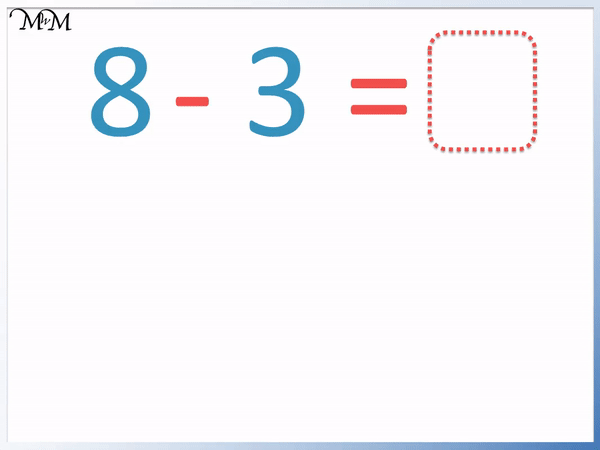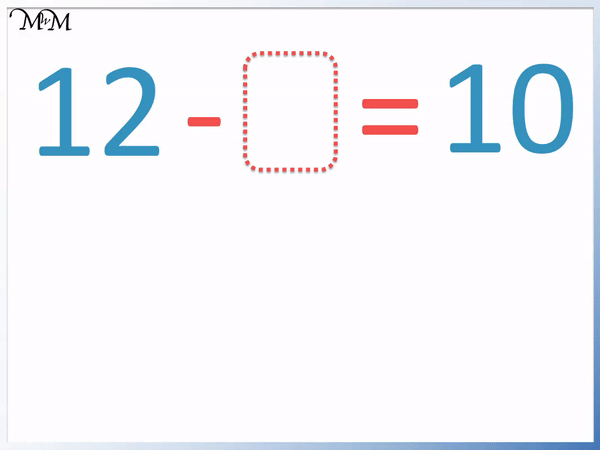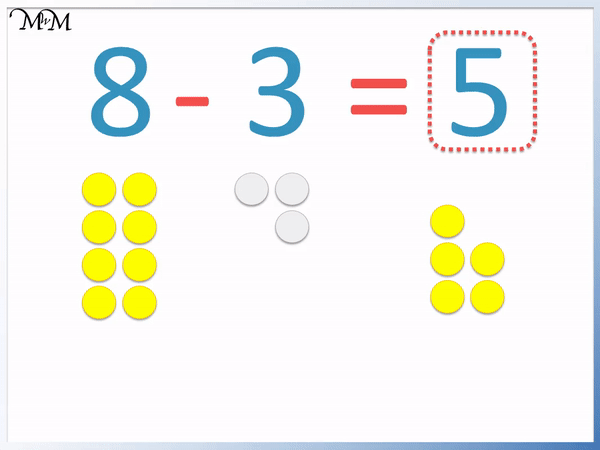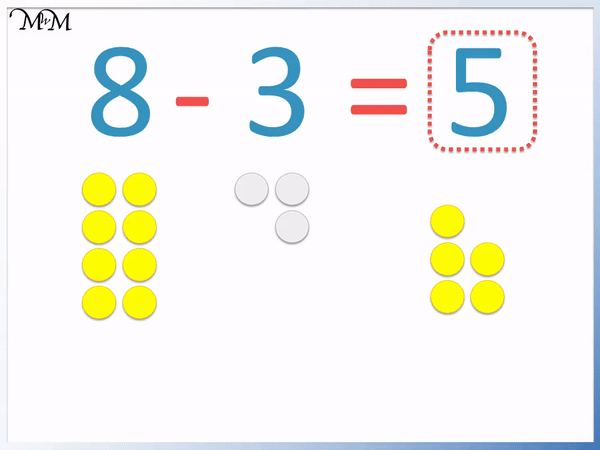# Subtraction Missing Numbers

Subtraction Missing Numbers• In this subtraction problem we have a missing number at the end of the subtraction sentence, after the equals sign.
• This means that the missing number is equal to 8 – 3.
• We work out the left hand side of the equals sign by subtracting 3 counters from the 8 counters.
• 8 – 3 = 5.
• If the missing number is after the equals sign then simply evaluate the subtraction before the equals sign.• In the original subtraction question we had 8 – 3 = 5.
• Below is the same subtraction but we are asked to fill in a missing number in a different position.
• To find the value of this missing number we think ‘which number subtract three equals five?’.
• From the previous question above we know the number is eight, however we can add the two other numbers together to obtain the answer.
• 5 + 3 = 8.• In this subtraction problem we are missing a number that is being subtracted.
• To fill in this subtraction missing number, we think ’12 subtract which number equals 10?’.
• We can subtract numbers from 12 until we reach 10.
• Starting from 12 we count down: 11 and then 10, which are 2 more numbers. We have subtracted 2.
• Alternatively we can subtract the other number from 12.
• 12 – 10 = 2.# How to Fill in Subtraction Missing Numbers

In this lesson we will be filling in missing numbers in subtraction problems.

We will be looking specifically at the typical questions encountered in primary school mathematics with three numbers in the complete subtraction sentence.

In these typical styles of subtraction missing number problems, there will be a largest number at the beginning of the subtraction, take away a smaller number. This will be equal to another number that is smaller than the first.

Below is a summary of the three cases of missing number problems that we may encounter.In summary, we have three cases of subtraction missing number problems that are solved as follows:

• If the missing number is immediately after the equals sign: Subtract the second number from the first.
• If the missing number is being subtracted from a larger number: Subtract the given answer from the larger number.
• If the missing number is immediately before the subtraction sign: Add the two other numbers together.

This summary is a quick guide to finding missing numbers in simple subtraction questions, however it is necessary to understand why this works and how to apply it.

We will consider the examples below to understand this further.

Our first example considers a direct subtraction of 8 take away 3.

Ideally we would recommend knowing basic subtraction facts very thoroughly as a prerequisite for these lessons.

We would aim to simply remember that since 3 + 5 = 8, then 8 – 3 = 5.

To practise this, we can teach the subtraction using counters as shown below.We can this teach simple subtraction by starting with the 8 counters, removing 3 and counting how many are left.This is the most common type of subtraction missing number question, in which the missing number is simply at the end of the number sentence, after the equals sign.The largest number that is immediately before the subtraction sign is 8.

We can see from the animation above, that the number directly before the subtraction sign is equal to the other two numbers added together.8 = 3 + 5

This can help us to work out missing numbers in further subtraction sentences.

Below is the same subtraction sentence, however this time the missing number is at the beginning, immediately in front of the subtraction sign.In this example we can easily see that if we know the answer to the original subtraction, then we can compare it with the missing number problem below it.

The first type of missing number problem is generally easier to solve, since it is written as an outright subtraction.

One way to solve subtraction missing number problems with a missing number before the equals sign is to compare it to a simple subtraction that you know.

We can see another example of this strategy below:7 – 5 = 2 is a routine subtraction and simply involves taking away five from seven to obtain the answer.

Children typically find the missing number problem below this to be a little more challenging.

It is asking ‘seven subtract what number gives an answer of five?’.

A simple strategy is to compare the question with the one above it: 7 – 5 = 2.

In order to do this, we actually need to remember the addition fact that 7 is made up of 2 + 5.

Knowing simple number facts such as these mean that the process is much easier as it helps to compare this missing number question with the one above it: 7 – 5 = 2.

We can see that an alternative to find the missing number of 7, is to add the two other numbers together.

2 + 5 = 7.

We can see that if the missing number in a subtraction is immediately before the subtraction sign ‘-‘, then we can add the number we are subtracting to the number after the equals sign to obtain the answer.

We will use this strategy of addition to fill in the following subtraction missing number more quickly and fluently.Since the missing number is immediately before the minus sign ‘-‘ then we can simply add the number we are subtracting to the number after the equals sign ‘=’.

7 + 3 = 10.

And so 10 – 3 = 7.

In the next example, we look at a subtraction with a missing number immediately after the subtraction sign ‘-‘ and before the equals sign ‘=’.The missing number is the answer to the question ’12 subtract what number equals 10?’.

We can start at 12 and count down until we reach 10.

After 12 we have: 11 and then 10, which is 2 more numbers.

12 – 2 = 10.

Again it helps to know subtraction facts such as these, however there is another trick we can use.

12 is the total. To fill in the missing number in this problem, we can subtract the other number from 12.

12 – 10 = 2.

We can work out a missing number immediately after a subtraction sign by taking away the number after the equals sign ‘=’ from the number immediately before the subtraction sign ‘-‘.

We will use this method to work out the missing number in our final subtraction example:The missing number will be the answer to the question ‘8 subtract what number equals 4?’.

We can subtract the other given number from 8 in order to find our answer.

8 – 4 = 4.

These techniques allow us to work out the three main styles of subtraction missing number problems, however it is important that a mastery of number order and a basic knowledge of addition and subtraction facts are understood first.

However, these problems can be a very good way of reinforcing these facts.Now try our lesson on Greater Than and Less Than Signs where we learn how to compare numbers.error: Content is protected !!